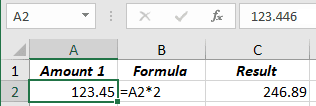# Different rounding effects in Excel

Excel
When you work with digits, it's crucial how you round them. It doesn't depend on what exactly you are rounding - decimals, thousands, or millions. You can see very different amounts after summing the figures, rounded in different ways:
• Rounded to an integer: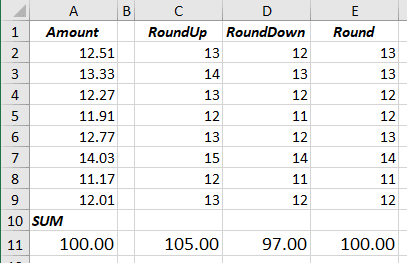• Rounded to two decimal digits: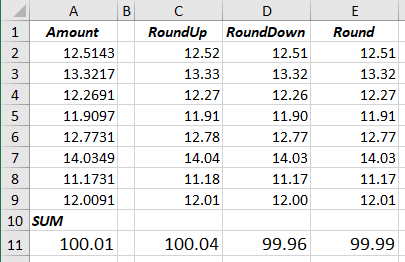1.   The functions for rounding: there are several different rounding functions in Excel:

= ROUND (<number>, <digits>)

= ROUNDUP (<number>, <digits>)

= ROUNDDOWN (<number>, <digits>)

Where:

• <number> is an amount to round,
• <digits> is a number of digits to round:
• If <digits> > 0, then <number> is rounded to the specified number of decimal places.
• If <digits> = 0, then <number> is rounded to the nearest integer.
• If <digits> < 0, then <number> is rounded to the left of the decimal point.

1.1.   The ROUND function rounds a number to a specified number of digits.

Rules of the rounding for ROUND function:

• the last kept digit shouldn't be changed if the first dropped digit is less than 5,
• the last kept digit should be increased by 1 if the first dropped digit is greater than 5.

For example: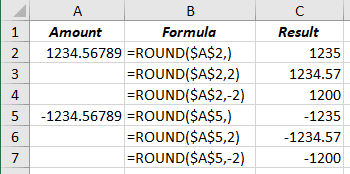1.2.   The ROUNDUP function always rounds the number up.

Rules of the rounding for ROUNDUP function:

• the last kept digit shouldn't be changed if the first dropped digit is 0 (zero),
• the last kept digit should be increased by 1 if the first dropped digit is greater than 0.

For example: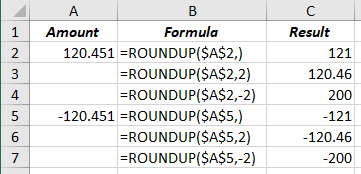1.3.   The ROUNDDOWN function always rounds a number down.

Rules of the rounding for ROUNDDOWN function:

• the last kept digit shouldn't be changed.

For example: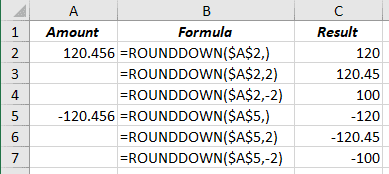2.   The order of rounding: rounding digits and summing them or summing digits and round the total amount.

For example: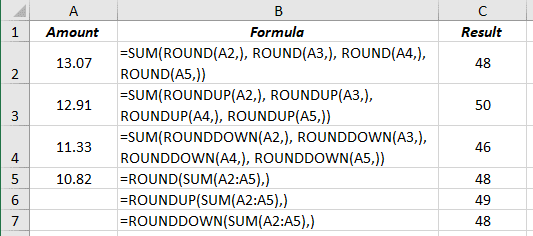3.   Besides the formulas, Excel can display digits in different ways.

Note: How Excel works with the amount in the cell, and its formatting is only a way how it displays the value, not how it calculates the value!

For example, using cell formatting, you can see a rounded amount and unexpected formula result: